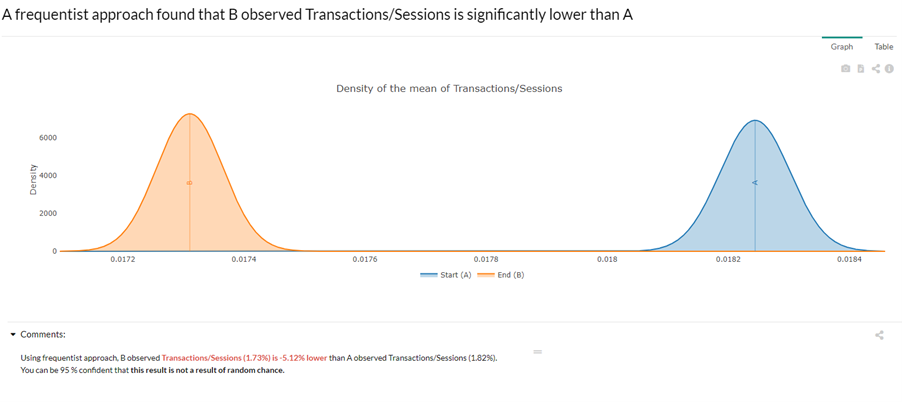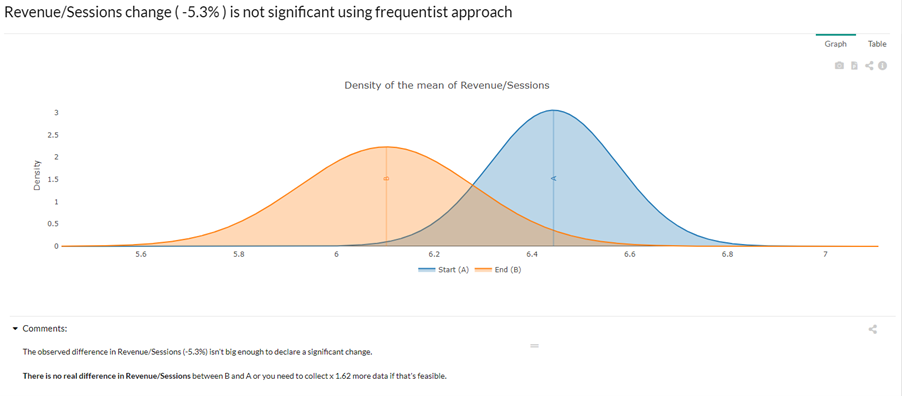# Assess - Frequentist model

### Frequentist Bernoulli

In the Bernoulli frequentist approach, the outcome has to be binary (1 or 0, e.g. Transactions). As long as this is true, any aggregation of the data can be used. The resulting chart shows the probability of distribution of the average value of the considered KPI.Behind the scenes: A frequentist Welch t-test is computed to test whether the mean outcome is significantly different between the Start and the End values of your comparison. The standard deviation used in the test is derived from the properties of the Bernoulli distribution. See also: wikipedia Test data: Gsheet

### Frequentist/ Other

In the frequentist (non bernoulli) approach, the outcome can be any variable. However, the data should be either unaggregated, or aggregated in comparable groups, so that DataMa can compute the actual variance of the success metric.

For instance, if you are measuring the statistical significance of the difference between two variants on a Average Basket Value, you need to have one line per transaction in your data source.

If the outcome variable is binary (e.g. Transaction) and data is aggregated, the Frequentist (Bernoulli) test should be used instead (for a precious gain of statistical power).

If you want to reduce the size of the dataset, and avoid having one line per occurrence, you can group them at a reasonable level and use the weight parameterBehind the scenes: A frequentist Welch t-test is computed to test whether the mean outcome is significantly different between the Start period and the End period. The standard deviation used in the test is derived from the properties of the sample (from the sample variance).